Image editing by painting a mask and specifying any text description to guide the image generation. TL;DR: Just train a StyleGAN / BigGAN generator and then, to edit an image region, just optimize the masked latent code using pretrained CLIP as a loss.

In this post, I will give a brief overview of the recent paper from MIT Paint by Word. Authors introduce a novel method which is to be able to paint in an image arbitrary new concepts described by text at any specific location provided by the user in a form of a mask. The proposed Paint by Word method can also generate a full image just based on a textual description.

TL;DR: Just train a StyleGAN / BigGAN generator and then, to edit an image region, just optimize the masked latent code using the similarity of the image and text in pretrained CLIP encoder space as a loss.

Point to a location in a synthesized image and apply an arbitrary new concept such as “rustic” or “opulent” or “happy dog.”. Then a new image can be generated (see image below).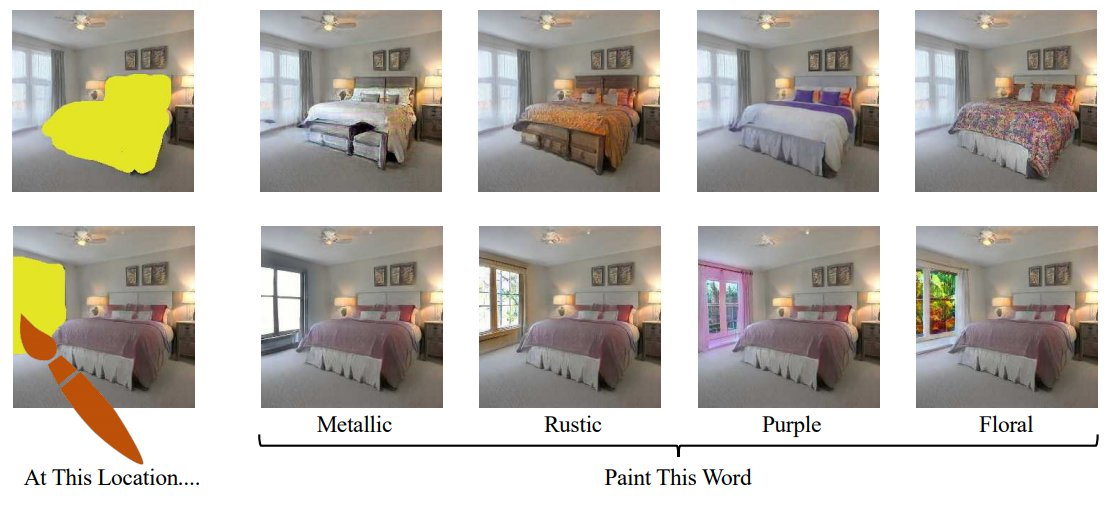## Method in a nutshell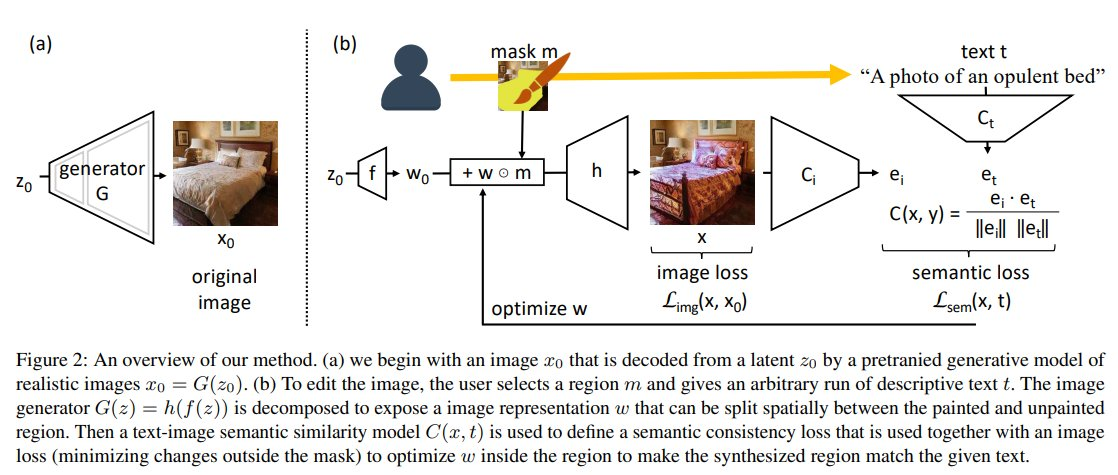### 🛠️ Two nets:

(1) a semantic similarity network $$C(x, t)$$ that scores the semantic consistency between an image $$x$$ and a text description $$t$$. It consists of two subnetworks: $$C_i(x)$$ which embeds images and $$C_t(t)$$ which embeds text.
(2) generative network $$G(z)$$ that is trained to synthesize realistic images given a random $$z$$; this network enforces realism.

We generate a realistic image $$G(z)$$ that matches descriptive text $$t$$ by optimizing

$z^∗ = arg min_z \mathcal{L}_{sem}(x, t) = arg min_z C(G(z), t)$ $\mathcal{L}_{sem}(x, t) = C(G(z), t) = \frac{C_i(G(z)) \cdot C_t(t)}{||C_i(G(z))|| \cdot ||C_t(t)||}$

To focus on changes in a local area, we direct the matching network $$C$$ to attend to only the region of the user’s brushstroke instead of the whole image. To do this we extract the latent representation $$w=f(z)$$ of the image and mask it using the user’s input and optimize only the masked region of the representation. In BigGAN experiments authors use the first convolutional block as $$f$$ and its output is then $$w=f(x)$$. In StyleGAN, $$w$$ is the style vector.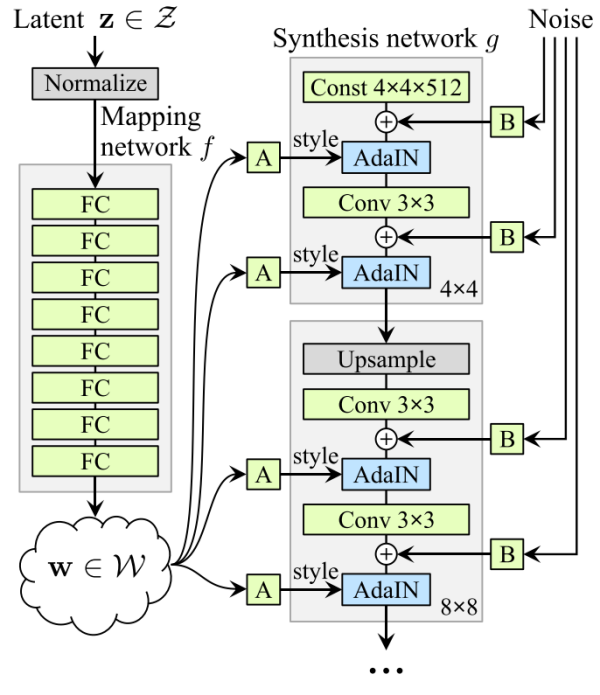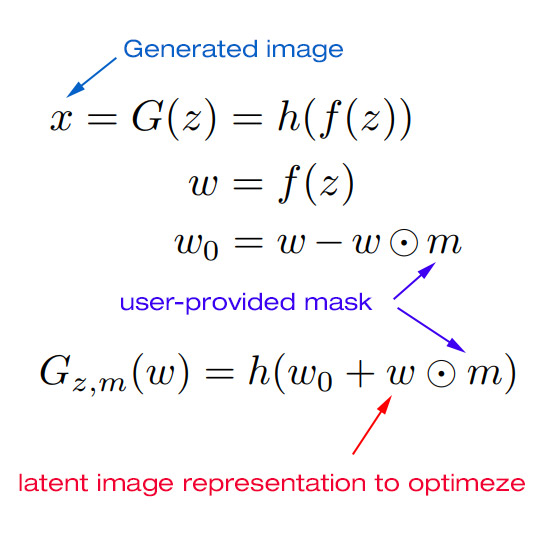To match the input textual description, we embed the output image $$x$$ and the text $$t$$ using networks $$C_i(x)$$ and $$C_t(t)$$ and maximize the similarity between these embeddings by backpropagating the gradients to the masked latent representation $$w \odot m$$, but not to $$w_0$$ (which corresponds to the region outside of the mask).

### Symmary of the used losses: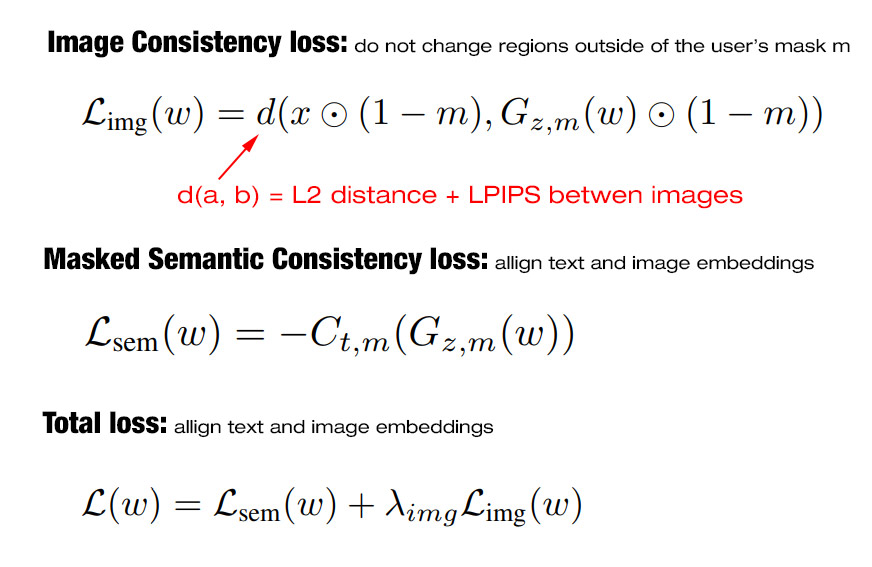Here is the loss ablation study. Masking the output image (c) vs masking the latent representation (d) for backprop. This shows that naive implementation is inferior to the proposed masking of the latent vector $$w$$.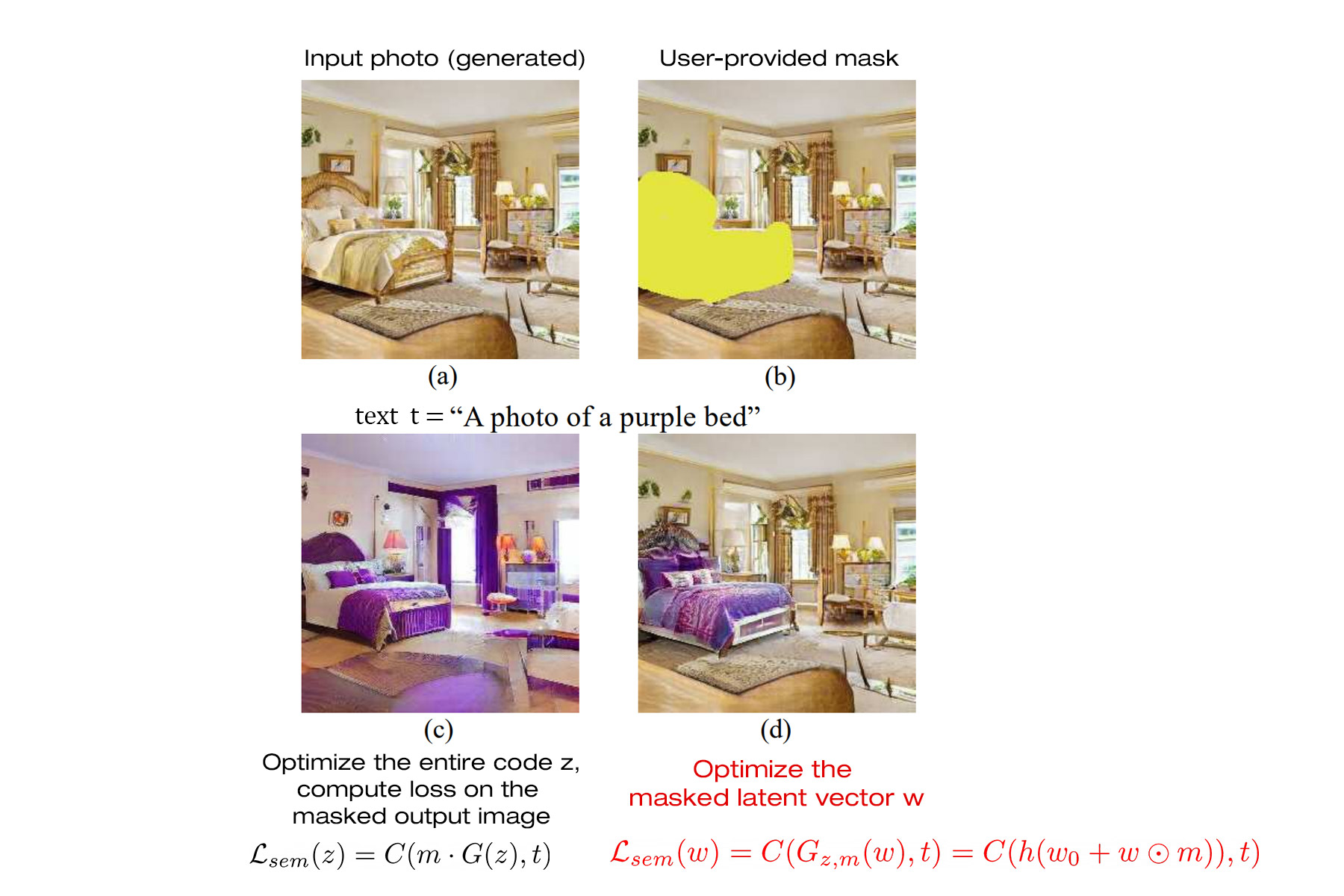## ⚔️ Full image generation. “Paint by Word” ⚔️ vs DALL-E

The proposed method has a simpler architecture than DALL-E and it does not explicitly train the generator to take textual description as input to the generator. The textual information comes only from the semantic loss.

For $$G$$ authors train a 256-pixel StyleGAN2 on the CUB dataset. And for $$C(x, t)$$ authors use an off-the-shelf CLIP model. The network is trained only on birds and it utterly fails to draw any other type of subject. Because of this narrow focus, it is unsurprising that it might be better at drawing realistic bird images than the DALL-E model, which is trained on a far broader variety of unconstrained images.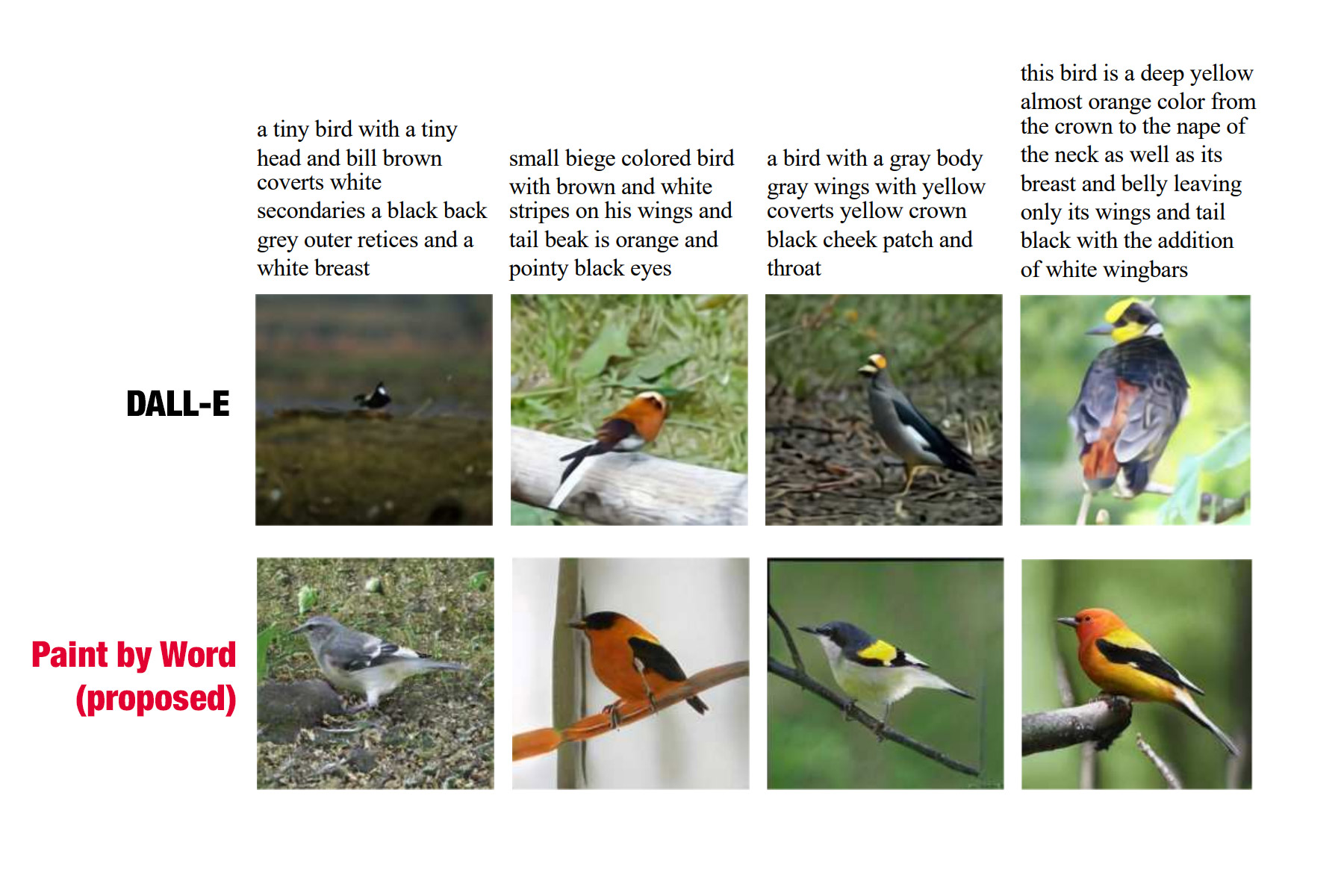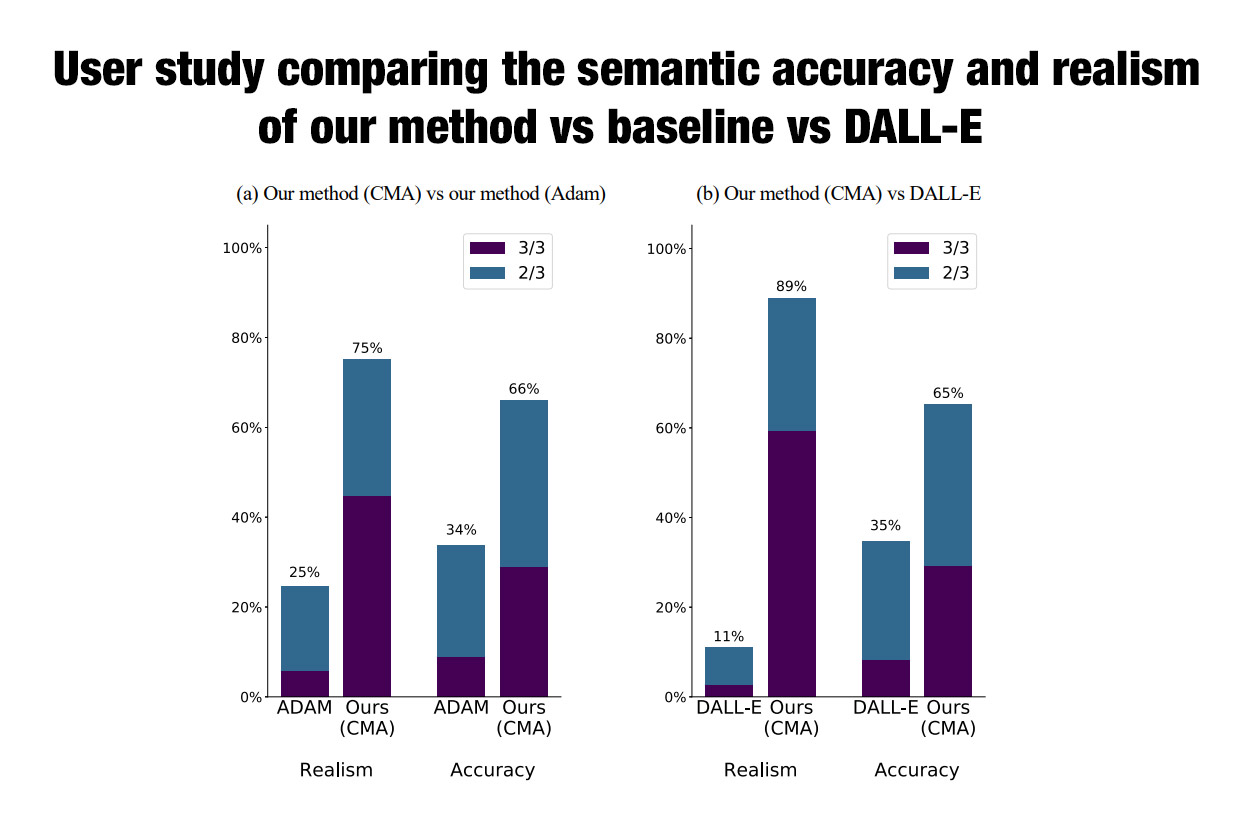Nevertheless, this experiment demonstrates that it is possible to obtain state-of-the-art semantic consistency, at least within a narrow image domain, without explicitly training the generator to take information about the textual concept as input.

Authors have also experimented with image editing using the BigGAN generator G(z) trained on ImageNet and Places: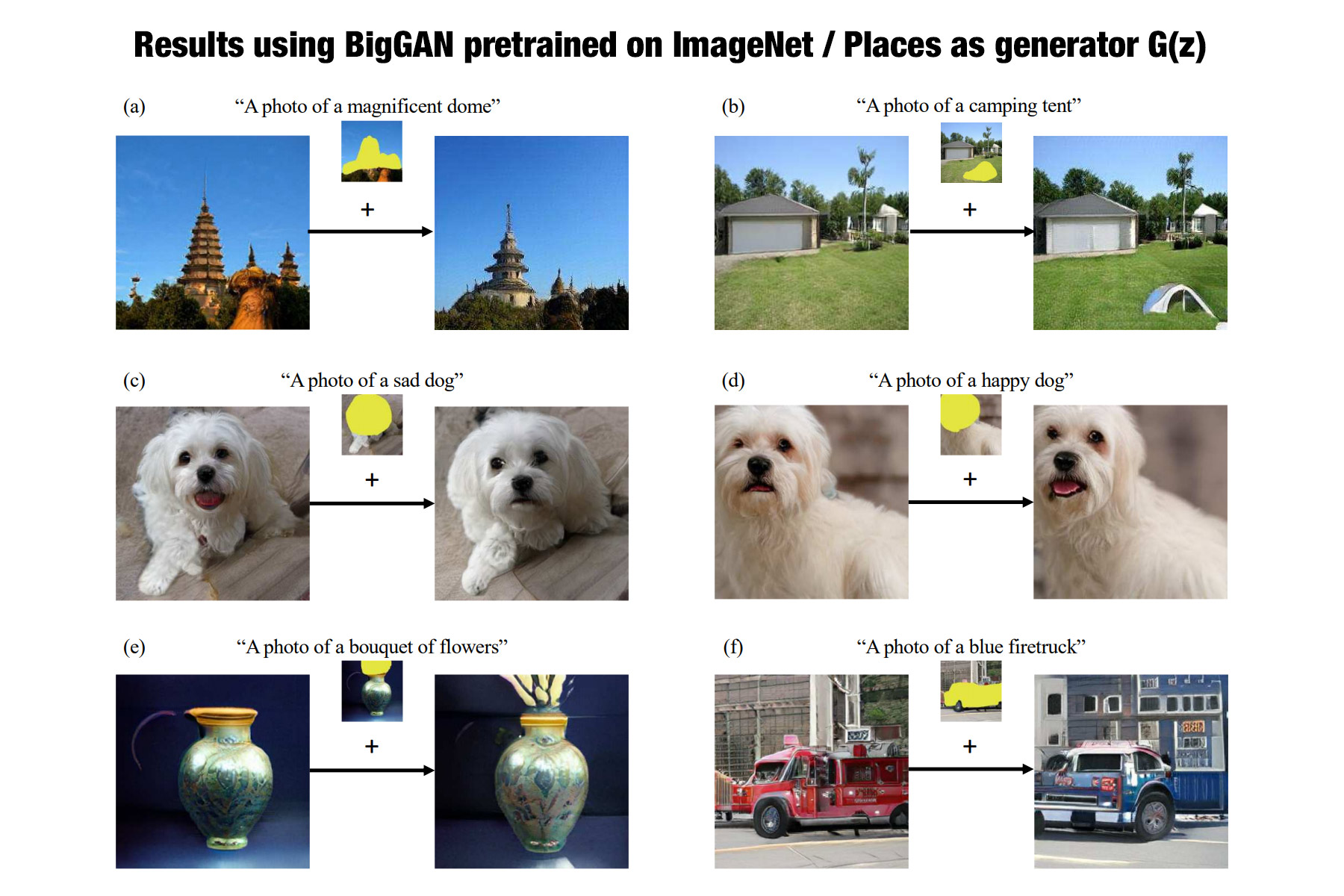• How to easily edit and compose images like in Photoshop using GANs. Recently, I did an overview of the paper “Using latent space regression to analyze and leverage compositionality in GANs”. This paper solves the following problem: Given an incomplete image or a collage of images, generate a realistic image. You can read a short blogpost here.

• BigSleep. Ryan Murdock has combined OpenAI’s CLIP and the generator from a BigGAN (read more in his blogpost). There is a repository BigSleep that wraps up his work and you can tune it on your own a GPU. In contrast to this paper, BigSleep cannot change only a region of the image and the entire image is modified according to the text prompt.

## ☑️ Conclusions

To conclude, this paper shows that even such a simple method can produce pretty amazing results.

🔥Just train your StyleGAN / BigGAN generator and then edit an image region just optimize the masked latent code using pretrained CLIP as a loss. That’s it!

### 📎 References:

📝 Arxiv paper: Paint By Word
🌐 Related blogpost: How to easily edit and compose images using GANs like in Photoshop

🌐 Related blogpost: DeepDaze, BigSleep, and Aleph2Image
⚒ GitHub: BigSleep

Feel free to ask me any questions in the comments below. Feedback is also very appreciated.

• Join my telegram channel not to miss other novel paper reviews like this!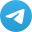@gradientdude
• Follow me on twitter@artsiom_s